# Selina Concise Chemistry Solution for ICSE Class 10 Chapter 3 Acids, Bases and Salts

Selina Publishers for class 10 Chemistry chapter 3 talks about the definition and properties of acids and bases. The chapter further deals with different reactions and students mainly learn how acids and bases react in different circumstances. They are also taught about real-world acids and their uses. Hence, students who want to get familiar with all the topics, equations and understand them clearly can access free concise Chemistry class 10 ICSE solutions for chapter 3 given here. The solutions can be downloaded easily and are available in the form of a PDF. Students can use them at their convenience to learn all the answers to difficult questions, understand and practice reactions and in the process be well prepared to write the exams.

## Selina Concise Chemistry Solution Class 10 Chapter 3 Acids, Bases and Salts:-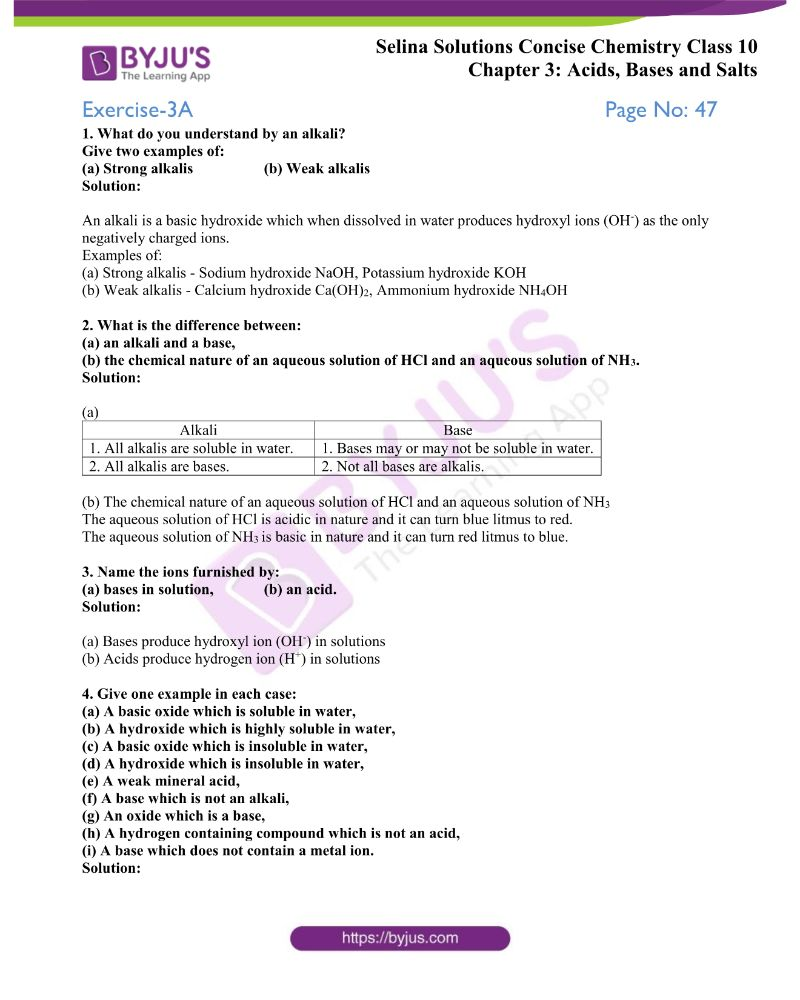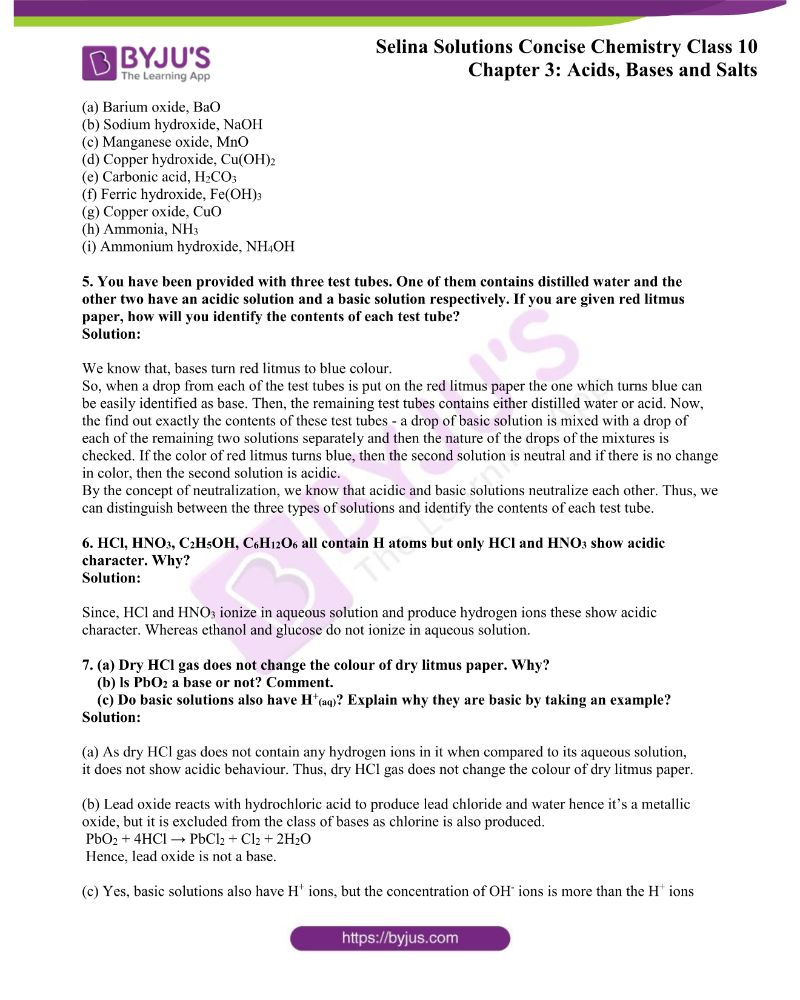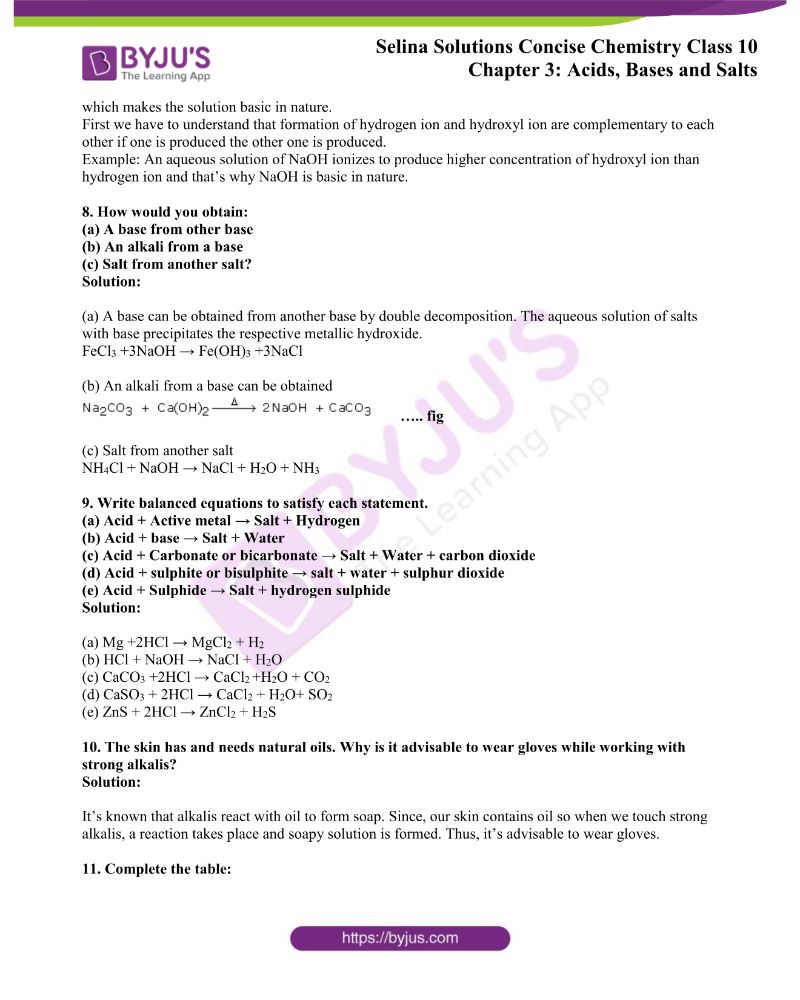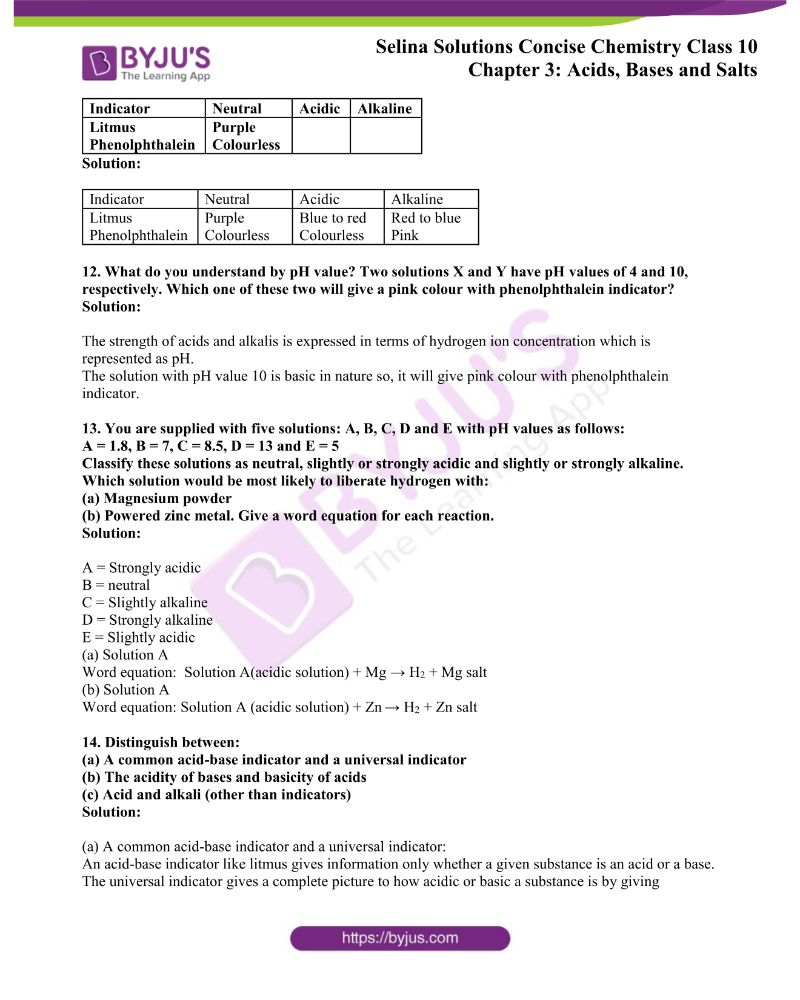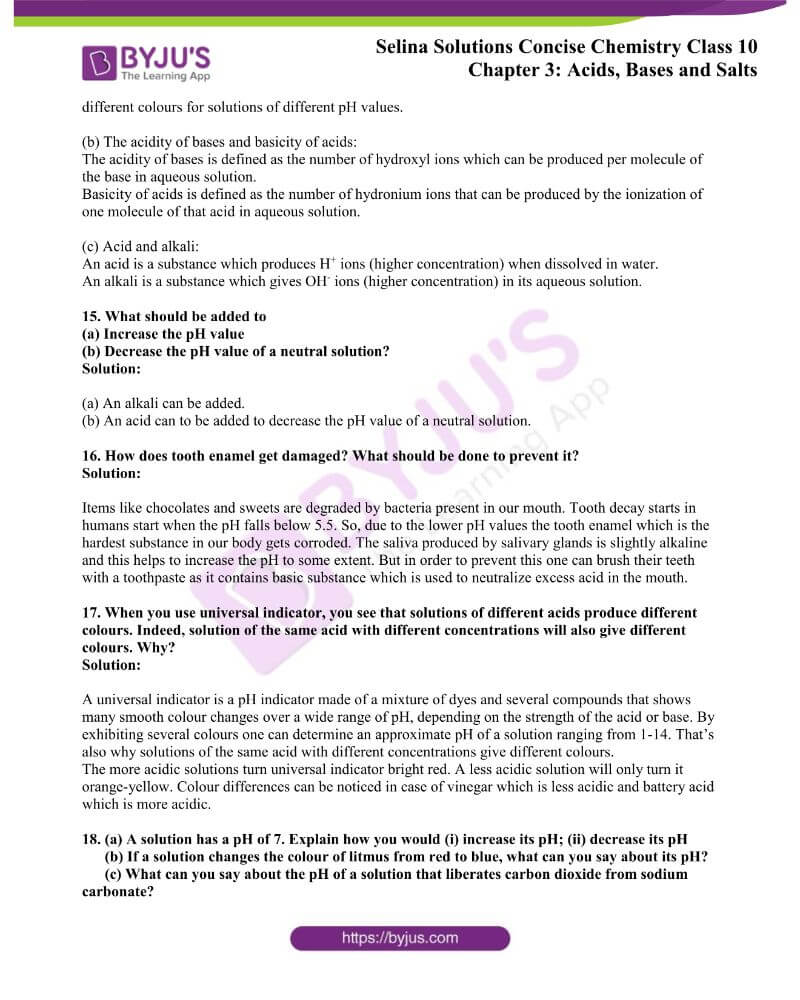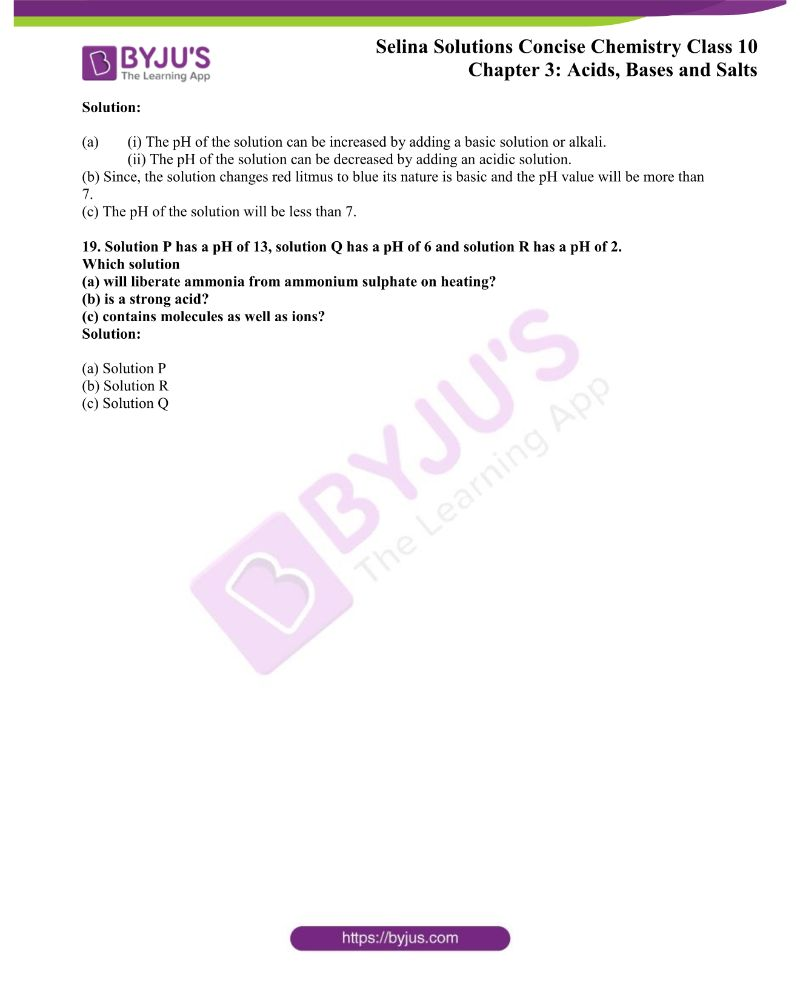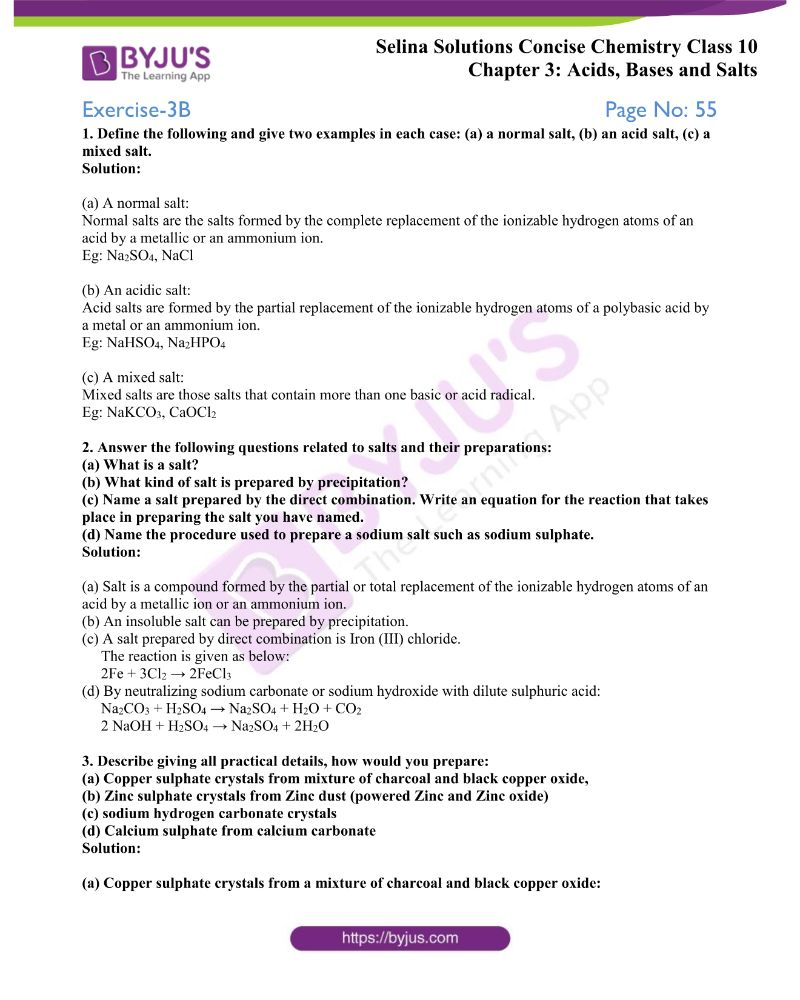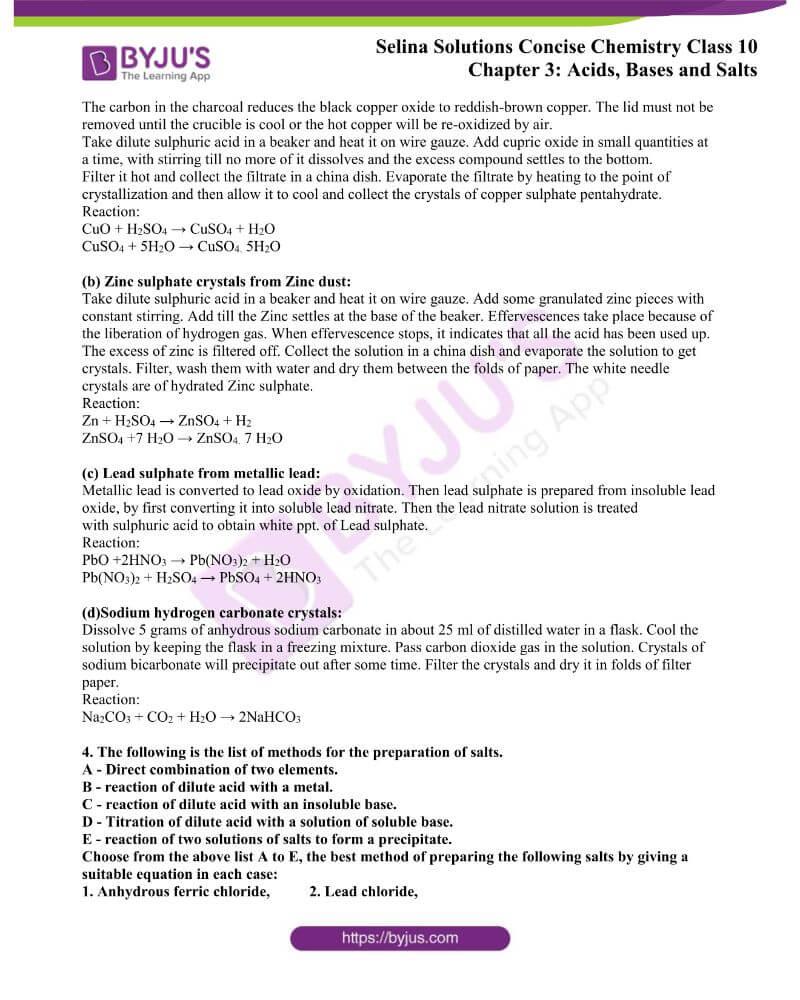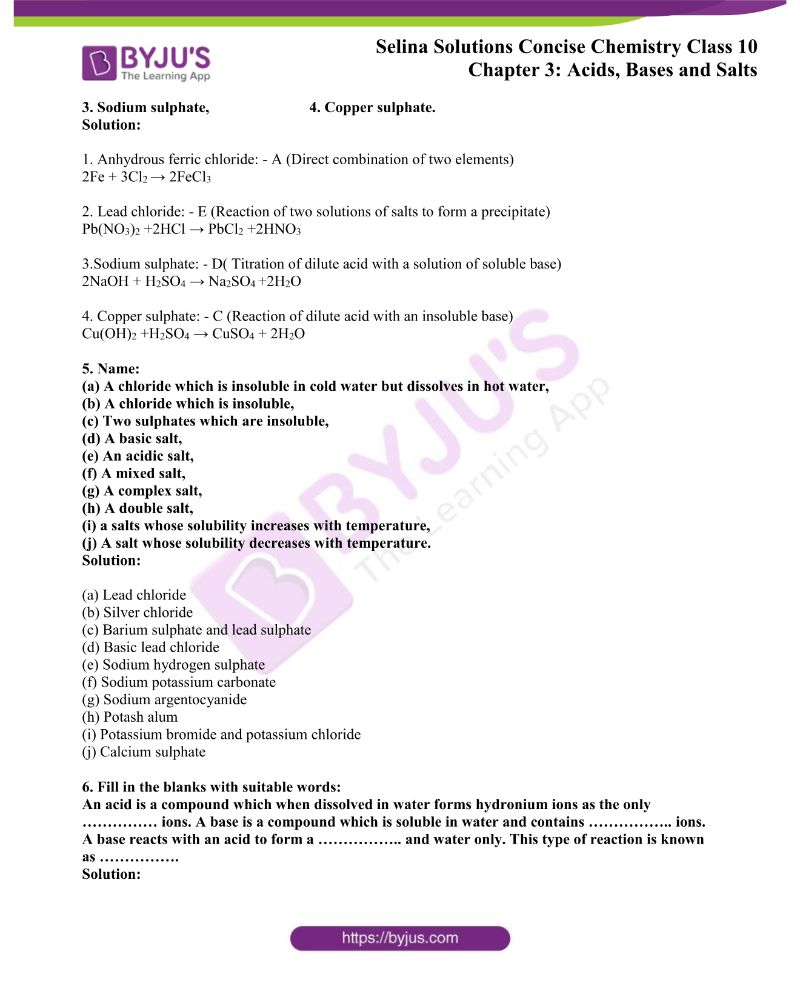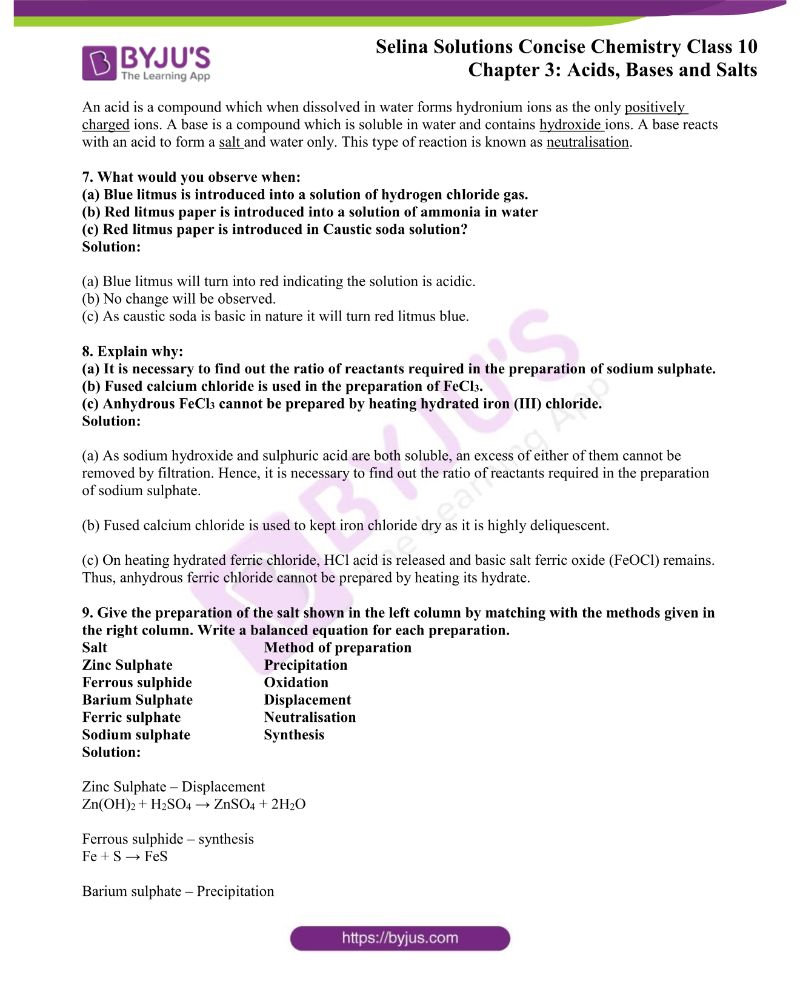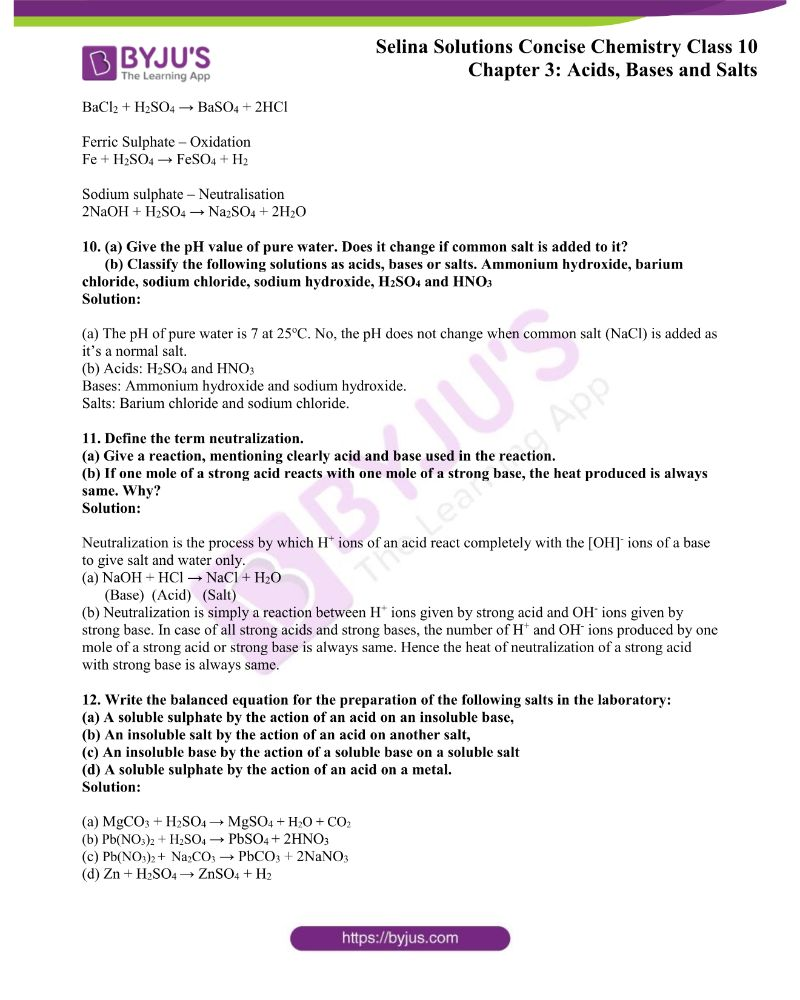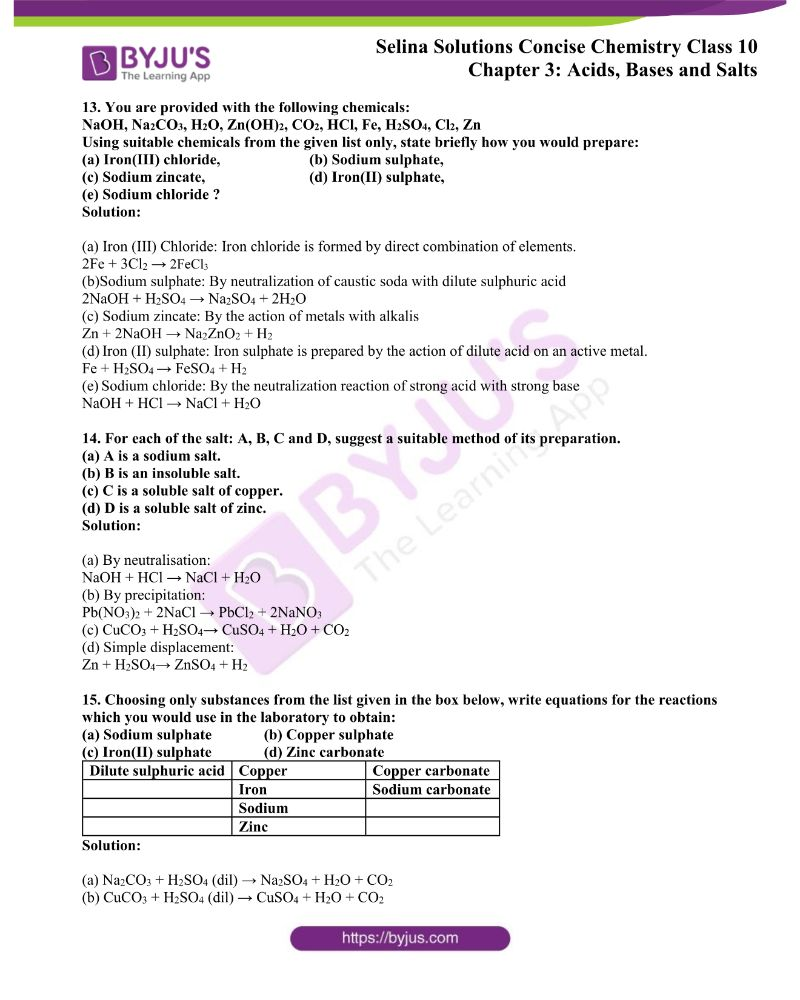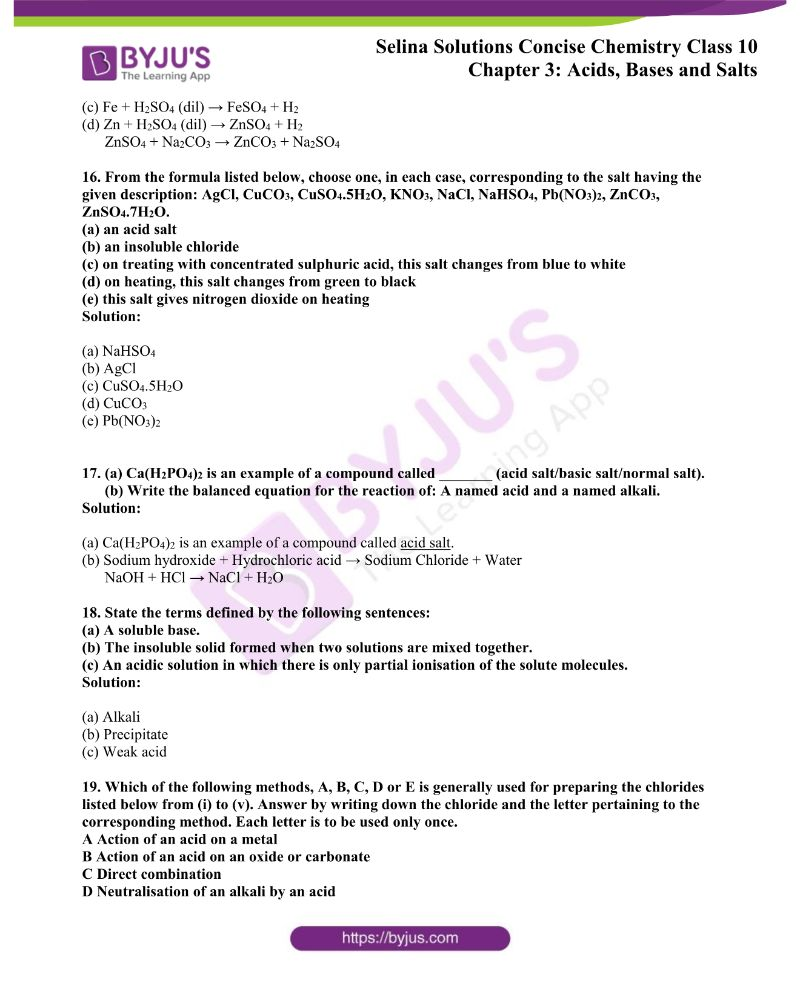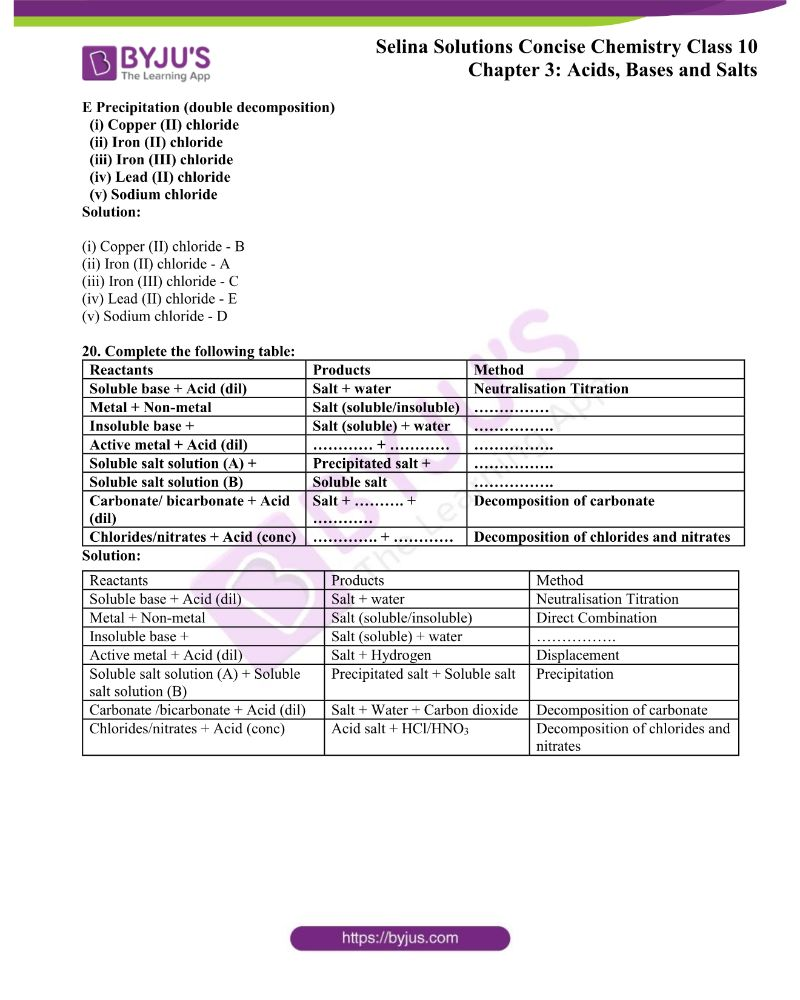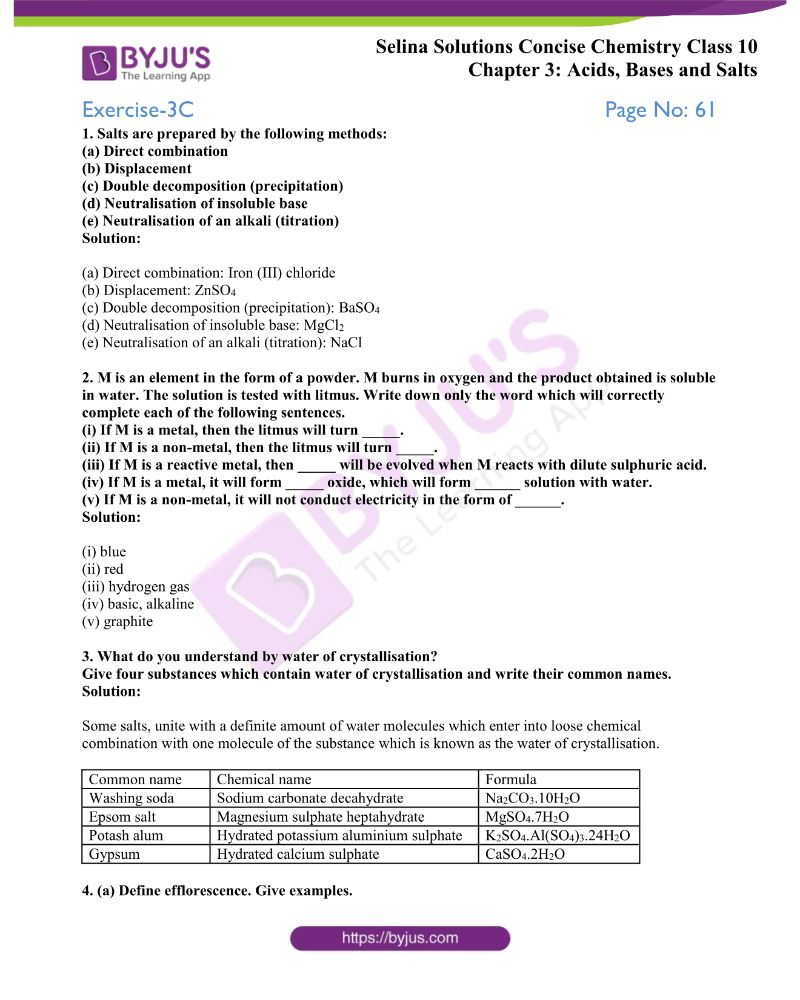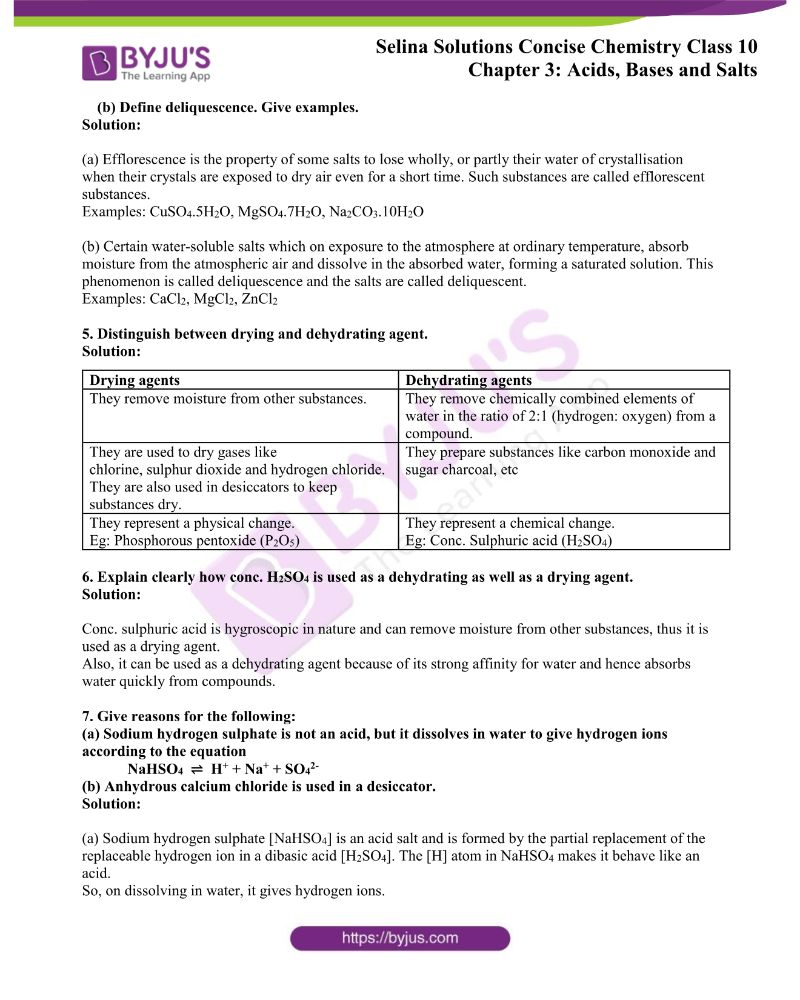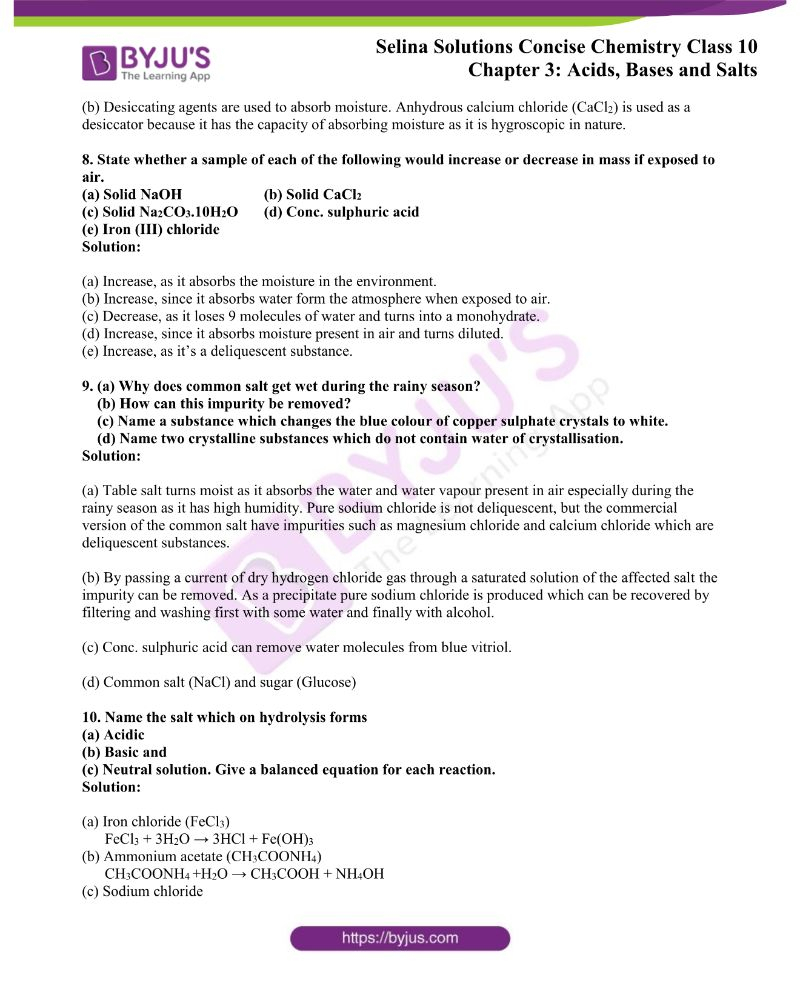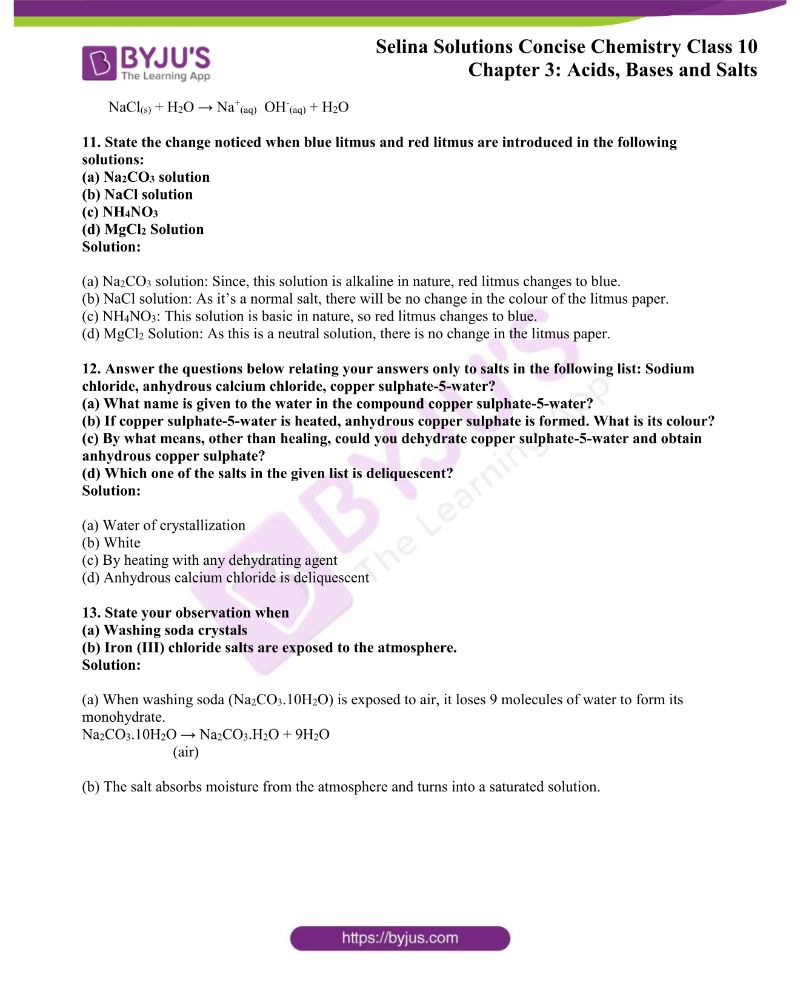### Access ICSE Class 10 Chemistry Chapter 3 Acids, Bases and Salts Solutions

Exercise-3A Page No: 47

1. What do you understand by an alkali?

Give two examples of:

(a) Strong alkalis (b) Weak alkalis

Solution:

An alkali is a basic hydroxide which when dissolved in water produces hydroxyl ions (OH) as the only negatively charged ions.

Examples of:

(a) Strong alkalis – Sodium hydroxide NaOH, Potassium hydroxide KOH

(b) Weak alkalis – Calcium hydroxide Ca(OH)2, Ammonium hydroxide NH4OH

2. What is the difference between:

(a) an alkali and a base,

(b) the chemical nature of an aqueous solution of HCl and an aqueous solution of NH3.

Solution:

(a)

 Alkali Base 1. All alkalis are soluble in water. 1. Bases may or may not be soluble in water. 2. All alkalis are bases. 2. Not all bases are alkalis.

(b) The chemical nature of an aqueous solution of HCl and an aqueous solution of NH3

The aqueous solution of HCl is acidic in nature and it can turn blue litmus to red.

The aqueous solution of NHis basic in nature and it can turn red litmus to blue.

3. Name the ions furnished by:

(a) bases in solution, (b) an acid.

Solution:

(a) Bases produce hydroxyl ion (OH) in solutions

(b) Acids produce hydrogen ion (H+) in solutions

4. Give one example in each case:

(a) A basic oxide which is soluble in water,

(b) A hydroxide which is highly soluble in water,

(c) A basic oxide which is insoluble in water,

(d) A hydroxide which is insoluble in water,

(e) A weak mineral acid,

(f) A base which is not an alkali,

(g) An oxide which is a base,

(h) A hydrogen-containing compound which is not an acid,

(i) A base which does not contain a metal ion.

Solution:

(a) Barium oxide, BaO

(b) Sodium hydroxide, NaOH

(c) Manganese oxide, MnO

(d) Copper hydroxide, Cu(OH)2

(e) Carbonic acid, H2CO3

(f) Ferric hydroxide, Fe(OH)3

(g) Copper oxide, CuO

(h) Ammonia, NH3

(i) Ammonium hydroxide, NH4OH

5. You have been provided with three test tubes. One of them contains distilled water and the other two have an acidic solution and a basic solution respectively. If you are given red litmus paper, how will you identify the contents of each test tube?

Solution:

We know that bases turn red litmus to blue colour.

So, when a drop from each of the test tubes is put on the red litmus paper the one which turns blue can be easily identified as a base. Then, the remaining test tubes contain either distilled water or acid. Now, the find out exactly the contents of these test tubes – a drop of basic solution is mixed with a drop of each of the remaining two solutions separately and then the nature of the drops of the mixtures is checked. If the colour of red litmus turns blue, then the second solution is neutral and if there is no change in colour, then the second solution is acidic.

By the concept of neutralization, we know that acidic and basic solutions neutralize each other. Thus, we can distinguish between the three types of solutions and identify the contents of each test tube.

6. HCl, HNO3, C2H5OH, C6H12O6 all contain H atoms but only HCl and HNO3 show acidic character. Why?

Solution:

Since, HCl and HNO3 ionize in aqueous solution and produce hydrogen ions these show acidic character. Whereas ethanol and glucose do not ionize in aqueous solution.

7. (a) Dry HCl gas does not change the colour of dry litmus paper. Why?

(b) ls PbO2 a base or not? Comment.

(c) Do basic solutions also have H+(aq)? Explain why they are basic by taking an example?

Solution:

(a) As dry HCl gas does not contain any hydrogen ions in it when compared to its aqueous solution, it does not show acidic behaviour. Thus, dry HCl gas does not change the colour of dry litmus paper.

(b) Lead oxide reacts with hydrochloric acid to produce lead chloride and water hence it’s a metallic oxide, but it is excluded from the class of bases as chlorine is also produced.

PbO2 + 4HCl → PbCl2 + Cl2 + 2H2O

Hence, lead oxide is not a base.

(c) Yes, basic solutions also have H+ ions, but the concentration of OH ions is more than the H+ ions which makes the solution basic in nature.

First, we have to understand that formation of hydrogen ion and hydroxyl ion are complementary to each other if one is produced the other one is produced.

Example: An aqueous solution of NaOH ionizes to produce a higher concentration of hydroxyl ion than hydrogen ion and that’s why NaOH is basic in nature.

8. How would you obtain:

(a) A base from another base

(b) An alkali from a base

(c) Salt from another salt?

Solution:

(a) A base can be obtained from another base by double decomposition. The aqueous solution of salts with base precipitates the respective metallic hydroxide.

FeCl3 +3NaOH → Fe(OH)3 +3NaCl

(b) An alkali from a base can be obtained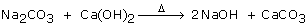….. fig

(c) Salt from another salt

NH4Cl + NaOH → NaCl + H2O + NH3

9. Write balanced equations to satisfy each statement.

(a) Acid + Active metal → Salt + Hydrogen

(b) Acid + base → Salt + Water

(c) Acid + Carbonate or bicarbonate → Salt + Water + carbon dioxide

(d) Acid + sulphite or bisulphite → salt + water + sulphur dioxide

(e) Acid + Sulphide → Salt + hydrogen sulphide

Solution:

(a) Mg +2HCl MgCl2 + H2

(b) HCl + NaOH NaCl + H2O

(c) CaCO3 +2HCl CaCl+H2O + CO2

(d) CaSO3 + 2HCl CaCl2 + H2O+ SO2

(e) ZnS + 2HCl ZnCl2 + H2S

10. The skin has and needs natural oils. Why is it advisable to wear gloves while working with strong alkalis?

Solution:

It’s known that alkalis react with oil to form soap. Since our skin contains oil so when we touch strong alkalis, a reaction takes place and a soapy solution is formed. Thus, it’s advisable to wear gloves.

11. Complete the table:

 Indicator Neutral Acidic Alkaline Litmus Phenolphthalein Purple Colourless

Solution:

 Indicator Neutral Acidic Alkaline Litmus Phenolphthalein Purple Colourless Blue to red Colourless Red to blue Pink

12. What do you understand by pH value? Two solutions X and Y have pH values of 4 and 10, respectively. Which one of these two will give a pink colour with a phenolphthalein indicator?

Solution:

The strength of acids and alkalis is expressed in terms of hydrogen ion concentration which is represented as pH.

The solution with pH value 10 is basic in nature so, it will give pink colour with phenolphthalein indicator.

13. You are supplied with five solutions: A, B, C, D and E with pH values as follows:

A = 1.8, B = 7, C = 8.5, D = 13 and E = 5

Classify these solutions as neutral, slightly or strongly acidic and slightly or strongly alkaline.

Which solution would be most likely to liberate hydrogen with:

(a) Magnesium powder

(b) Powered zinc metal. Give a word equation for each reaction.

Solution:

A = Strongly acidic

B = neutral

C = Slightly alkaline

D = Strongly alkaline

E = Slightly acidic

(a) Solution A

Word equation: Solution A(acidic solution) + Mg H2 + Mg salt

(b) Solution A

Word equation: Solution A (acidic solution) + Zn H2 + Zn salt

14. Distinguish between:

(a) A common acid-base indicator and a universal indicator

(b) The acidity of bases and basicity of acids

(c) Acid and alkali (other than indicators)

Solution:

(a) A common acid-base indicator and a universal indicator:

An acid-base indicator like litmus gives information only whether a given substance is an acid or a base. The universal indicator gives a complete picture to how acidic or basic a substance is by giving different colours for solutions of different pH values.

(b) The acidity of bases and basicity of acids:

The acidity of bases is defined as the number of hydroxyl ions which can be produced per molecule of the base in aqueous solution.

Basicity of acids is defined as the number of hydronium ions that can be produced by the ionization of one molecule of that acid in aqueous solution.

(c) Acid and alkali:

An acid is a substance which produces H+ ions (higher concentration) when dissolved in water.

An alkali is a substance which gives OH ions (higher concentration) in its aqueous solution.

15. What should be added to

(a) Increase the pH value

(b) Decrease the pH value of a neutral solution?

Solution:

(a) An alkali can be added.

(b) An acid can to be added to decrease the pH value of a neutral solution.

16. How does tooth enamel get damaged? What should be done to prevent it?

Solution:

Items like chocolates and sweets are degraded by bacteria present in our mouth. Tooth decay starts in humans start when the pH falls below 5.5. So, due to the lower pH values, the tooth enamel which is the hardest substance in our body gets corroded. The saliva produced by salivary glands is slightly alkaline and this helps to increase the pH to some extent. But in order to prevent this one can brush their teeth with toothpaste as it contains a basic substance which is used to neutralize excess acid in the mouth.

17. When you use universal indicator, you see that solutions of different acids produce different colours. Indeed, the solution of the same acid with different concentrations will also give different colours. Why?

Solution:

A universal indicator is a pH indicator made of a mixture of dyes and several compounds that shows many smooth colour changes over a wide range of pH, depending on the strength of the acid or base. By exhibiting several colours one can determine an approximate pH of a solution ranging from 1-14. That’s also why solutions of the same acid with different concentrations give different colours.

The more acidic solutions turn universal indicator bright red. A less acidic solution will only turn it orange-yellow. Colour differences can be noticed in case of vinegar which is less acidic and battery acid which is more acidic.

18. (a) A solution has a pH of 7. Explain how you would (i) increase its pH; (ii) decrease its pH

(b) If a solution changes the colour of litmus from red to blue, what can you say about its pH?

(c) What can you say about the pH of a solution that liberates carbon dioxide from sodium carbonate?

Solution:

(a) (i) The pH of the solution can be increased by adding a basic solution or alkali.

(ii) The pH of the solution can be decreased by adding an acidic solution.

(b) Since, the solution changes red litmus to blue its nature is basic and the pH value will be more than 7.

(c) The pH of the solution will be less than 7.

19. Solution P has a pH of 13, solution Q has a pH of 6 and solution R has a pH of 2.

Which solution

(a) will liberate ammonia from ammonium sulphate on heating?

(b) is a strong acid?

(c) contains molecules as well as ions?

Solution:

(a) Solution P

(b) Solution R

(c) Solution Q

Exercise-3B Page No: 55

1. Define the following and give two examples in each case: (a) a normal salt, (b) an acid salt, (c) a mixed salt.

Solution:

(a) A normal salt:

Normal salts are the salts formed by the complete replacement of the ionizable hydrogen atoms of an acid by a metallic or an ammonium ion.

Eg: Na2SO4, NaCl

(b) An acidic salt:

Acid salts are formed by the partial replacement of the ionizable hydrogen atoms of a polybasic acid by a metal or an ammonium ion.

Eg: NaHSO4, Na2HPO4

(c) A mixed salt:

Mixed salts are those salts that contain more than one basic or acid radical.

Eg: NaKCO3, CaOCl2

2. Answer the following questions related to salts and their preparations:

(a) What is salt?

(b) What kind of salt is prepared by precipitation?

(c) Name a salt prepared by the direct combination. Write an equation for the reaction that takes place in preparing the salt you have named.

(d) Name the procedure used to prepare a sodium salt such as sodium sulphate.

Solution:

(a) Salt is a compound formed by the partial or total replacement of the ionizable hydrogen atoms of an acid by a metallic ion or an ammonium ion.

(b) An insoluble salt can be prepared by precipitation.

(c) A salt prepared by direct combination is Iron (III) chloride.

The reaction is given as below:

2Fe + 3Cl2 → 2FeCl3

(d) By neutralizing sodium carbonate or sodium hydroxide with dilute sulphuric acid:

Na2CO3 + H2SO4 → Na2SO4 + H2O + CO2

2 NaOH + H2SO4 → Na2SO4 + 2H2O

3. Describe giving all practical details, how would you prepare:

(a) Copper sulphate crystals from a mixture of charcoal and black copper oxide,

(b) Zinc sulphate crystals from Zinc dust (powered Zinc and Zinc oxide)

(c) sodium hydrogen carbonate crystals

(d) Calcium sulphate from calcium carbonate

Solution:

(a) Copper sulphate crystals from a mixture of charcoal and black copper oxide:

The carbon in the charcoal reduces the black copper oxide to reddish-brown copper. The lid must not be removed until the crucible is cool or the hot copper will be re-oxidized by air.

Take dilute sulphuric acid in a beaker and heat it on wire gauze. Add cupric oxide in small quantities at a time, with stirring till no more of it dissolves and the excess compound settles to the bottom.

Filter it hot and collect the filtrate in a china dish. Evaporate the filtrate by heating to the point of crystallization and then allow it to cool and collect the crystals of copper sulphate pentahydrate.

Reaction:

CuO + H2SO4 → CuSO4 + H2O

CuSO4 + 5H2O → CuSO4. 5H2O

(b) Zinc sulphate crystals from Zinc dust:

Take dilute sulphuric acid in a beaker and heat it on wire gauze. Add some granulated zinc pieces with constant stirring. Add till the Zinc settles at the base of the beaker. Effervescences take place because of the liberation of hydrogen gas. When effervescence stops, it indicates that all the acid has been used up. The excess of zinc is filtered off. Collect the solution in a china dish and evaporate the solution to get crystals. Filter, wash them with water and dry them between the folds of paper. The white needle crystals are of hydrated Zinc sulphate.

Reaction:

Zn + H2SO4 → ZnSO4 + H2

ZnSO4 +7 H2O → ZnSO4. 7 H2O

Metallic lead is converted to lead oxide by oxidation. Then lead sulphate is prepared from insoluble lead oxide, by first converting it into soluble lead nitrate. Then the lead nitrate solution is treated with sulphuric acid to obtain white ppt. of Lead sulphate.

Reaction:

PbO +2HNO3 → Pb(NO3)2 + H2O

Pb(NO3)2 + H2SO4 → PbSO4 + 2HNO3

(d)Sodium hydrogen carbonate crystals:

Dissolve 5 grams of anhydrous sodium carbonate in about 25 ml of distilled water in a flask. Cool the solution by keeping the flask in a freezing mixture. Pass carbon dioxide gas in the solution. Crystals of sodium bicarbonate will precipitate out after some time. Filter the crystals and dry it in folds of filter paper.

Reaction:

Na2CO3 + CO2 + H2O → 2NaHCO3

4. The following is the list of methods for the preparation of salts.

A – Direct combination of two elements.

B – a reaction of dilute acid with a metal.

C – a reaction of dilute acid with an insoluble base.

D – Titration of dilute acid with a solution of soluble base.

E – a reaction of two solutions of salts to form a precipitate.

Choose from the above list A to E, the best method of preparing the following salts by giving a suitable equation in each case:

1. Anhydrous ferric chloride, 2. Lead chloride,

3. Sodium sulphate, 4. Copper sulphate.

Solution:

1. Anhydrous ferric chloride: – A (Direct combination of two elements)

2Fe + 3Cl2 → 2FeCl3

2. Lead chloride: – E (Reaction of two solutions of salts to form a precipitate)

Pb(NO3)2 +2HCl → PbCl2 +2HNO3

3.Sodium sulphate: – D( Titration of dilute acid with a solution of soluble base)

2NaOH + H2SO4 → Na2SO+2H2O

4. Copper sulphate: – C (Reaction of dilute acid with an insoluble base)

Cu(OH)2 +H2SO4 → CuSO4 + 2H2O

5. Name:

(a) A chloride which is insoluble in cold water but dissolves in hot water,

(b) A chloride which is insoluble,

(c) Two sulphates which are insoluble,

(d) A basic salt,

(e) An acidic salt,

(f) A mixed salt,

(g) A complex salt,

(h) A double salt,

(i) salts whose solubility increases with temperature,

(j) A salt whose solubility decreases with temperature.

Solution:

(b) Silver chloride

(c) Barium sulphate and lead sulphate

(e) Sodium hydrogen sulphate

(f) Sodium potassium carbonate

(g) Sodium argentocyanide

(h) Potash alum

(i) Potassium bromide and potassium chloride

(j) Calcium sulphate

6. Fill in the blanks with suitable words:

An acid is a compound which when dissolved in water forms hydronium ions as the only …………… ions. A base is a compound which is soluble in water and contains …………….. ions. A base reacts with an acid to form a …………….. and water only. This type of reaction is known as …………….

Solution:

An acid is a compound which when dissolved in water forms hydronium ions as the only positively charged ions. A base is a compound which is soluble in water and contains hydroxide ions. A base reacts with an acid to form a salt and water only. This type of reaction is known as neutralisation.

7. What would you observe when:

(a) Blue litmus is introduced into a solution of hydrogen chloride gas.

(b) Red litmus paper is introduced into a solution of ammonia in water

(c) Red litmus paper is introduced in Caustic soda solution?

Solution:

(a) Blue litmus will turn into red indicating the solution is acidic.

(b) No change will be observed.

(c) As caustic soda is basic in nature it will turn red litmus blue.

8. Explain why:

(a) It is necessary to find out the ratio of reactants required in the preparation of sodium sulphate.

(b) Fused calcium chloride is used in the preparation of FeCl3.

(c) Anhydrous FeCl3 cannot be prepared by heating hydrated iron (III) chloride.

Solution:

(a) As sodium hydroxide and sulphuric acid are both soluble, an excess of either of them cannot be removed by filtration. Hence, it is necessary to find out the ratio of reactants required in the preparation of sodium sulphate.

(b) Fused calcium chloride is used to kept iron chloride dry as it is highly deliquescent.

(c) On heating hydrated ferric chloride, HCl acid is released and basic salt ferric oxide (FeOCl) remains. Thus, anhydrous ferric chloride cannot be prepared by heating its hydrate.

9. Give the preparation of the salt shown in the left column by matching with the methods given in the right column. Write a balanced equation for each preparation.

Salt Method of preparation

Zinc Sulphate Precipitation

Ferrous sulphide Oxidation

Barium Sulphate Displacement

Ferric sulphate Neutralisation

Sodium sulphate Synthesis

Solution:

Zinc Sulphate – Displacement

Zn(OH)2 + H2SO4 → ZnSO4 + 2H2O

Ferrous sulphide – synthesis

Fe + S → FeS

Barium sulphate – Precipitation

BaCl2 + H2SO4 → BaSO4 + 2HCl

Ferric Sulphate – Oxidation

Fe + H2SO4 → FeSO4 + H2

Sodium sulphate – Neutralisation

2NaOH + H2SO4 → Na2SO4 + 2H2O

10. (a) Give the pH value of pure water. Does it change if common salt is added to it?

(b) Classify the following solutions as acids, bases or salts. Ammonium hydroxide, barium chloride, sodium chloride, sodium hydroxide, H2SO4 and HNO3

Solution:

(a) The pH of pure water is 7 at 25oC. No, the pH does not change when common salt (NaCl) is added as it’s a normal salt.

(b) Acids: H2SO4 and HNO3

Bases: Ammonium hydroxide and sodium hydroxide.

Salts: Barium chloride and sodium chloride.

11. Define the term neutralization.

(a) Give a reaction, mentioning clearly acid and base used in the reaction.

(b) If one mole of a strong acid reacts with one mole of a strong base, the heat produced is always same. Why?

Solution:

Neutralization is the process by which H+ ions of an acid react completely with the [OH] ions of a base to give salt and water only.

(a) NaOH + HCl → NaCl + H2O

(Base) (Acid) (Salt)

(b) Neutralization is simply a reaction between H+ ions given by strong acid and OH ions given by a strong base. In case of all strong acids and strong bases, the number of H+ and OH ions produced by one mole of a strong acid or strong base is always the same. Hence the heat of neutralization of a strong acid with a strong base is always the same.

12. Write the balanced equation for the preparation of the following salts in the laboratory:

(a) A soluble sulphate by the action of an acid on an insoluble base,

(b) An insoluble salt by the action of an acid on another salt,

(c) An insoluble base by the action of a soluble base on a soluble salt

(d) A soluble sulphate by the action of an acid on a metal.

Solution:

(a) MgCO3 + H2SO4 → MgSO4 + H2O + CO2

(b) Pb(NO3)2 + H2SO4 → PbSO4 + 2HNO3

(c) Pb(NO3)2 + Na2CO3 → PbCO3 + 2NaNO3

(d) Zn + H2SO4 → ZnSO4 + H2

13. You are provided with the following chemicals:

NaOH, Na2CO3, H2O, Zn(OH)2, CO2, HCl, Fe, H2SO4, Cl2, Zn

Using suitable chemicals from the given list only, state briefly how you would prepare:

(a) Iron(III) chloride, (b) Sodium sulphate,

(c) Sodium zincate, (d) Iron(II) sulphate,

(e) Sodium chloride ?

Solution:

(a) Iron (III) Chloride: Iron chloride is formed by direct combination of elements.

2Fe + 3Cl2 → 2FeCl3

(b)Sodium sulphate: By neutralization of caustic soda with dilute sulphuric acid

2NaOH + H2SO4 → Na2SO4 + 2H2O

(c) Sodium zincate: By the action of metals with alkalis

Zn + 2NaOH → Na2ZnO2 + H2

(d) Iron (II) sulphate: Iron sulphate is prepared by the action of dilute acid on an active metal.

Fe + H2SO4 → FeSO4 + H2

(e) Sodium chloride: By the neutralization reaction of a strong acid with a strong base

NaOH + HCl → NaCl + H2O

14. For each of the salt: A, B, C and D, suggest a suitable method of its preparation.

(a) A is a sodium salt.

(b) B is an insoluble salt.

(c) C is a soluble salt of copper.

(d) D is a soluble salt of zinc.

Solution:

(a) By neutralisation:

NaOH + HCl → NaCl + H2O

(b) By precipitation:

Pb(NO3)2 + 2NaCl → PbCl2 + 2NaNO3

(c) CuCO3 + H2SO4→ CuSO4 + H2O + CO2

(d) Simple displacement:

Zn + H2SO4→ ZnSO4 + H2

15. Choosing only substances from the list given in the box below, write equations for the reactions which you would use in the laboratory to obtain:

(a) Sodium sulphate (b) Copper sulphate

(c) Iron(II) sulphate (d) Zinc carbonate

 Dilute sulphuric acid Copper Copper carbonate Iron Sodium carbonate Sodium Zinc

Solution:

(a) Na2CO3 + H2SO4 (dil) → Na2SO4 + H2O + CO2

(b) CuCO3 + H2SO4 (dil) → CuSO4 + H2O + CO2

(c) Fe + H2SO4 (dil) → FeSO4 + H2

(d) Zn + H2SO4 (dil) → ZnSO4 + H2

ZnSO4 + Na2CO3 → ZnCO3 + Na2SO4

16. From the formula listed below, choose one, in each case, corresponding to the salt having the given description: AgCl, CuCO3, CuSO4.5H2O, KNO3, NaCl, NaHSO4, Pb(NO3)2, ZnCO3, ZnSO4.7H2O.

(a) an acid salt

(b) an insoluble chloride

(c) on treating with concentrated sulphuric acid, this salt changes from blue to white

(d) on heating, this salt changes from green to black

(e) this salt gives nitrogen dioxide on heating

Solution:

(a) NaHSO4

(b) AgCl

(c) CuSO4.5H2O

(d) CuCO3

(e) Pb(NO3)2

17. (a) Ca(H2PO4)2 is an example of a compound called _______ (acid salt/basic salt/normal salt).

(b) Write the balanced equation for the reaction of: A named acid and a named alkali.

Solution:

(a) Ca(H2PO4)2 is an example of a compound called acid salt.

(b) Sodium hydroxide + Hydrochloric acid → Sodium Chloride + Water

NaOH + HCl → NaCl + H2O

18. State the terms defined by the following sentences:

(a) A soluble base.

(b) The insoluble solid formed when two solutions are mixed together.

(c) An acidic solution in which there is only partial ionisation of the solute molecules.

Solution:

(a) Alkali

(b) Precipitate

(c) Weak acid

19. Which of the following methods, A, B, C, D or E is generally used for preparing the chlorides listed below from (i) to (v). Answer by writing down the chloride and the letter pertaining to the corresponding method. Each letter is to be used only once.

A Action of an acid on a metal

B Action of acid on an oxide or carbonate

C Direct combination

D Neutralisation of alkali by an acid

E Precipitation (double decomposition)

(i) Copper (II) chloride

(ii) Iron (II) chloride

(iii) Iron (III) chloride

(v) Sodium chloride

Solution:

(i) Copper (II) chloride – B

(ii) Iron (II) chloride – A

(iii) Iron (III) chloride – C

(iv) Lead (II) chloride – E

(v) Sodium chloride – D

20. Complete the following table:

 Reactants Products Method Soluble base + Acid (dil) Salt + water Neutralisation Titration Metal + Non-metal Salt (soluble/insoluble) …………… Insoluble base + Salt (soluble) + water ……………. Active metal + Acid (dil) ………… + ………… ……………. Soluble salt solution (A) + Precipitated salt + ……………. Soluble salt solution (B) Soluble salt ……………. Carbonate/ bicarbonate + Acid (dil) Salt + ………. + ………… Decomposition of carbonate Chlorides/nitrates + Acid (conc) …………. + ………… Decomposition of chlorides and nitrates

Solution:

 Reactants Products Method Soluble base + Acid (dil) Salt + water Neutralisation Titration Metal + Non-metal Salt (soluble/insoluble) Direct Combination Insoluble base + Salt (soluble) + water ……………. Active metal + Acid (dil) Salt + Hydrogen Displacement Soluble salt solution (A) + Soluble salt solution (B) Precipitated salt + Soluble salt Precipitation Carbonate /bicarbonate + Acid (dil) Salt + Water + Carbon dioxide Decomposition of carbonate Chlorides/nitrates + Acid (conc) Acid salt + HCl/HNO3 Decomposition of chlorides and nitrates

Exercise-3C Page No: 61

1. Salts are prepared by the following methods:

(a) Direct combination

(b) Displacement

(c) Double decomposition (precipitation)

(d) Neutralisation of insoluble base

(e) Neutralisation of an alkali (titration)

Solution:

(a) Direct combination: Iron (III) chloride

(b) Displacement: ZnSO4

(c) Double decomposition (precipitation): BaSO4

(d) Neutralisation of insoluble base: MgCl2

(e) Neutralisation of an alkali (titration): NaCl

2. M is an element in the form of a powder. M burns in oxygen and the product obtained is soluble in water. The solution is tested with litmus. Write down only the word which will correctly complete each of the following sentences.

(i) If M is a metal, then the litmus will turn _____.

(ii) If M is a non-metal, then the litmus will turn _____.

(iii) If M is a reactive metal, then _____ will be evolved when M reacts with dilute sulphuric acid.

(iv) If M is a metal, it will form _____ oxide, which will form ______ solution with water.

(v) If M is a non-metal, it will not conduct electricity in the form of ______.

Solution:

(i) blue

(ii) red

(iii) hydrogen gas

(iv) basic, alkaline

(v) graphite

3. What do you understand by the water of crystallisation?

Give four substances which contain water of crystallisation and write their common names.

Solution:

Some salts, unite with a definite amount of water molecules which enter into loose chemical combination with one molecule of the substance which is known as the water of crystallisation.

 Common name Chemical name Formula Washing soda Sodium carbonate decahydrate Na2CO3.10H2O Epsom salt Magnesium sulphate heptahydrate MgSO4.7H2O Potash alum Hydrated potassium aluminium sulphate K2SO4.Al(SO4)3.24H2O Gypsum Hydrated calcium sulphate CaSO4.2H2O

4. (a) Define efflorescence. Give examples.

(b) Define deliquescence. Give examples.

Solution:

(a) Efflorescence is the property of some salts to lose wholly, or partly their water of crystallisation when their crystals are exposed to dry air even for a short time. Such substances are called efflorescent substances.

Examples: CuSO4.5H2O, MgSO4.7H2O, Na2CO3.10H2O

(b) Certain water-soluble salts which on exposure to the atmosphere at ordinary temperature, absorb moisture from the atmospheric air and dissolve in the absorbed water, forming a saturated solution. This phenomenon is called deliquescence and the salts are called deliquescent.

Examples: CaCl2, MgCl2, ZnCl2

5. Distinguish between drying and dehydrating agent.

Solution:

 Drying agents Dehydrating agents They remove moisture from other substances. They remove chemically combined elements of water in the ratio of 2:1 (hydrogen: oxygen) from a compound. They are used to dry gases like chlorine, sulphur dioxide and hydrogen chloride. They are also used in desiccators to keep substances dry. They prepare substances like carbon monoxide and sugar charcoal, etc They represent physical change. Eg: Phosphorous pentoxide (P2O5) They represent a chemical change. Eg: Conc. Sulphuric acid (H2SO4)

6. Explain clearly how conc. H2SO4 is used as a dehydrating as well as a drying agent.

Solution:

Conc. sulphuric acid is hygroscopic in nature and can remove moisture from other substances, thus it is used as a drying agent.

Also, it can be used as a dehydrating agent because of its strong affinity for water and hence absorbs water quickly from compounds.

7. Give reasons for the following:

(a) Sodium hydrogen sulphate is not an acid, but it dissolves in water to give hydrogen ions according to the equation

NaHSO4 ⇌  H+ + Na+ + SO42-

(b) Anhydrous calcium chloride is used in a desiccator.

Solution:

(a) Sodium hydrogen sulphate [NaHSO4] is an acid salt and is formed by the partial replacement of the replaceable hydrogen ion in a dibasic acid [H2SO4]. The [H] atom in NaHSO4 makes it behave like an acid.

So, on dissolving in water, it gives hydrogen ions.

(b) Desiccating agents are used to absorb moisture. Anhydrous calcium chloride (CaCl2) is used as a desiccator because it has the capacity of absorbing moisture as it is hygroscopic in nature.

8. State whether a sample of each of the following would increase or decrease in mass if exposed to air.

(a) Solid NaOH (b) Solid CaCl2

(c) Solid Na2CO3.10H2O (d) Conc. sulphuric acid

(e) Iron (III) chloride

Solution:

(a) Increase, as it absorbs the moisture in the environment.

(b) Increase, since it absorbs water from the atmosphere when exposed to air.

(c) Decrease, as it loses 9 molecules of water and turns into a monohydrate.

(d) Increase, since it absorbs moisture present in the air and turns diluted.

(e) Increase, as it’s a deliquescent substance.

9. (a) Why does common salt get wet during the rainy season?

(b) How can this impurity be removed?

(c) Name a substance which changes the blue colour of copper sulphate crystals to white.

(d) Name two crystalline substances which do not contain water of crystallisation.

Solution:

(a) Table salt turns moist as it absorbs the water and water vapour present in the air, especially during the rainy season as it has high humidity. Pure sodium chloride is not deliquescent, but the commercial version of the common salt has impurities such as magnesium chloride and calcium chloride which are deliquescent substances.

(b) By passing a current of dry hydrogen chloride gas through a saturated solution of the affected salt the impurity can be removed. As a precipitate pure sodium chloride is produced which can be recovered by filtering and washing first with some water and finally with alcohol.

(c) Conc. sulphuric acid can remove water molecules from blue vitriol.

(d) Common salt (NaCl) and sugar (Glucose)

10. Name the salt which on hydrolysis forms

(a) Acidic

(b) Basic and

(c) Neutral solution. Give a balanced equation for each reaction.

Solution:

(a) Iron chloride (FeCl3)

FeCl3 + 3H2O → 3HCl + Fe(OH)3

(b) Ammonium acetate (CH3COONH4)

CH3COONH+H2O → CH3COOH + NH4OH

(c) Sodium chloride

NaCl(s) + H2O → Na+(aq)  OH(aq) + H2O

11. State the change noticed when blue litmus and red litmus are introduced in the following solutions:

(a) Na2CO3 solution

(b) NaCl solution

(c) NH4NO3

(d) MgCl2 Solution

Solution:

(a) Na2CO3 solution: Since, this solution is alkaline in nature, red litmus changes to blue.

(b) NaCl solution: As it’s a normal salt, there will be no change in the colour of the litmus paper.

(c) NH4NO3: This solution is basic in nature, so red litmus changes to blue.

(d) MgCl2 Solution: As this is a neutral solution, there is no change in the litmus paper.

12. Answer the questions below relating your answers only to salts in the following list: Sodium chloride, anhydrous calcium chloride, copper sulphate-5-water?

(a) What name is given to the water in the compound copper sulphate-5-water?

(b) If copper sulphate-5-water is heated, anhydrous copper sulphate is formed. What is its colour?

(c) By what means, other than healing, could you dehydrate copper sulphate-5-water and obtain anhydrous copper sulphate?

(d) Which one of the salts in the given list is deliquescent?

Solution:

(a) Water of crystallization

(b) White

(c) By heating with any dehydrating agent

(d) Anhydrous calcium chloride is deliquescent

(a) Washing soda crystals

(b) Iron (III) chloride salts are exposed to the atmosphere.

Solution:

(a) When washing soda (Na2CO3.10H2O) is exposed to air, it loses 9 molecules of water to form its monohydrate.

Na2CO3.10H2O → Na2CO3.H2O + 9H2O

(air)

(b) The salt absorbs moisture from the atmosphere and turns into a saturated solution.

The given solutions are as per the 2019-20 Concise Selina textbook. The Selina Solutions for the academic year 2023-24 will be updated soon.